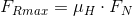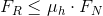## Static friction coefficient

The maximum hold force (static friction) FR caused by adhesive friction depends on normal force FN and the static friction coefficient µH (for the correct values, see the corresponding tables). This results in the general formulae as specified in eq. 1 and eq. 2:

Eq. 1Eq. 2FRmax     = maximum hold force in Newton [N]
µH     = Static friction coefficient
FN     = Normal force in Newton [N]
FR     = Hold force in Newton [N]

The equations allow for calculation of the maximum hold force with the static friction coefficient and the normal force given, and with an inequation resulting for static friction FR.